# a. How much heat (kJ), at 1 atm, is required to raise the temperature of 25.0...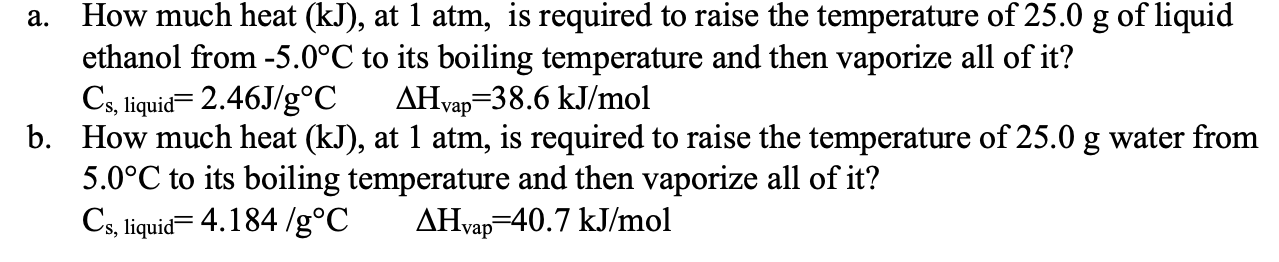a. How much heat (kJ), at 1 atm, is required to raise the temperature of 25.0 g of liquid ethanol from -5.0°C to its boiling temperature and then vaporize all of it? Cs, liquid= 2.46J/g°C AHvap=38.6 kJ/mol b. How much heat (kJ), at 1 atm, is required to raise the temperature of 25.0 g water from 5.0°C to its boiling temperature and then vaporize all of it? Cs, liquid=4.184 /g°C AHvap=40.7 kJ/mol

This Homework Help Question: "a. How much heat (kJ), at 1 atm, is required to raise the temperature of 25.0..." No answers yet.

We need 10 more requests to produce the answer to this homework help question. Share with your friends to get the answer faster!

0 /10 have requested the answer to this homework help question.

Once 10 people have made a request, the answer to this question will be available in 1-2 days.
All students who have requested the answer will be notified once they are available.

#### Earn Coin

Coins can be redeemed for fabulous gifts.

Similar Homework Help Questions
• ### How much heat (in kJ) is required to raise the temperature of 202.8 g of ethanol...

How much heat (in kJ) is required to raise the temperature of 202.8 g of ethanol (mw=46.07) from 40.34 °C to its boiling point of  78.37 °C and then vaporize it completely creating ethanol gas at the boiling temperature? (specific heat = 2.46 J/g°C, ΔHvap = 48.6 kJ/mol)

• ### How much heat (in kJ) is required to raise the temperature of 288.7 g of ethanol...

How much heat (in kJ) is required to raise the temperature of 288.7 g of ethanol (mw=46.07) from 39.38 °C to its boiling point of 78.37 °C and then vaporize it completely creating ethanol gas at the boiling temperature? (specific heat = 2.46 J/g°C, ΔHvap = 48.6 kJ/mol)

• ### How much heat (in kJ) is required to raise the temperature of 124.9 g of ethanol...

How much heat (in kJ) is required to raise the temperature of 124.9 g of ethanol (mw=46.07) from 30.24 °C to its boiling point of  78.37 °C and then vaporize it completely creating ethanol gas at the boiling temperature? (specific heat = 2.46 J/g°C, ΔHvap = 48.6 kJ/mol)

• ### QUESTION 3 How much heat (in kJ) is required to raise the temperature of 122 g of ethanol (mw 46.07) from 11.29 °C to i...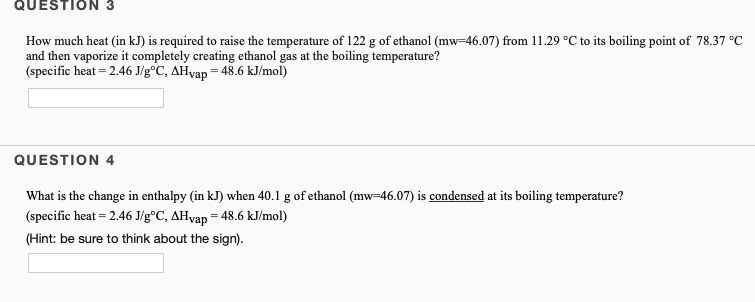QUESTION 3 How much heat (in kJ) is required to raise the temperature of 122 g of ethanol (mw 46.07) from 11.29 °C to its boiling point of 78.37 °C and then vaporize it completely creating ethanol gas at the boiling temperature? (specific heat - 2.46 J/g°C, AHyap = 48.6 kJ/mol) QUESTION 4 What is the change in enthalpy (in kJ) when 40.1 g of ethanol (mw-46.07) is condensed at its boiling temperature? (specific heat 2.46 J/g°C, AHvap 48.6 kJ/mol)...

• ### QUESTION 3 How much heat (in kJ) is required to raise the temperature of 141,4 g...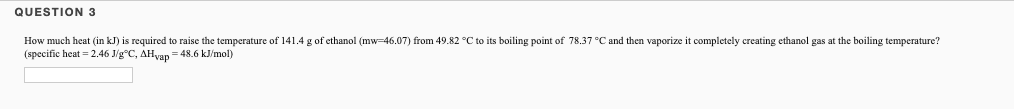QUESTION 3 How much heat (in kJ) is required to raise the temperature of 141,4 g of ethanol (mw-46,07) from 49.82 °C to its boiling point of 78.37 °C and then vaporize it completely creating ethanol gas at the boiling temperature? (specific heat=2.46 J/gºC, AH yap = 48.6 kJ/mol)

• ### Calculate the amount of heat (in kJ) required to raise the temperature of 14.0 g of...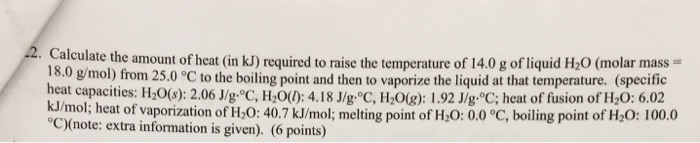Calculate the amount of heat (in kJ) required to raise the temperature of 14.0 g of liquid H_2O (molar mass = 18.0 g/mol) from 25.0 degree C to the boiling point and then to vaporize the liquid at that temperature, (specific heal capacities: H_2O(s): 2.06 J/g degree C, H_2O(l): 4.18 J/g degree C, H_2O(g): 1.92 J/g degree C; heat of fusion of H_2O: 6.02 kJ/mol; heat of vaporization of H_2O: 40.7 kJ/mol; melting point of H_2O: 0.0 degree C, boiling...

• ### Given the following information for ethanol, C,H,OH (at 1 atm), calculate the amount of heat in kneeded (at 1 atm)...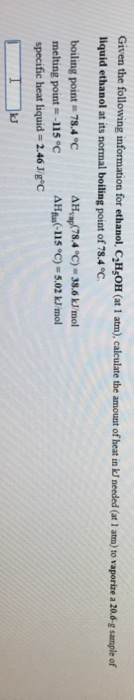Given the following information for ethanol, C,H,OH (at 1 atm), calculate the amount of heat in kneeded (at 1 atm) to vaporize a 20.6-g sample of liquid ethanol at its normal boiling point of 78.4 °C. boiling point = 78.4 °C AHvap 78.4 °C) = 38.6 kJ/mol melting point = -115°C AH(-115 °C) -5.02 kJ/mol specific heat liquid = 2.46 J/gºC

• ### How much energy is required to raise the temperature of 33.6 g of water from -25.5°C to 86.4°C

How much energy is required to raise the temperature of 33.6 g of water from -25.5°C to 86.4°C?melting point 0.0°C boiling point 100.0°C specific heat solid 2.05 J/g · °C specfic heat liquid 4.184 J/g · °C specific heat gas 2.02 J/g · °C H° fusion 6.02 kJ/mol H° vaporization 40.7 kJ/mol

• ### How much heat is required to vaporize 100.0 g of ethanol, C2H,OH, at its boiling point?...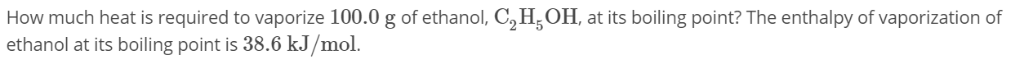How much heat is required to vaporize 100.0 g of ethanol, C2H,OH, at its boiling point? The enthalpy of vaporization of ethanol at its boiling point is 38.6 kJ/mol.

• ### 1. How much heat is required at constant pressure to melt 1 mole of ice at...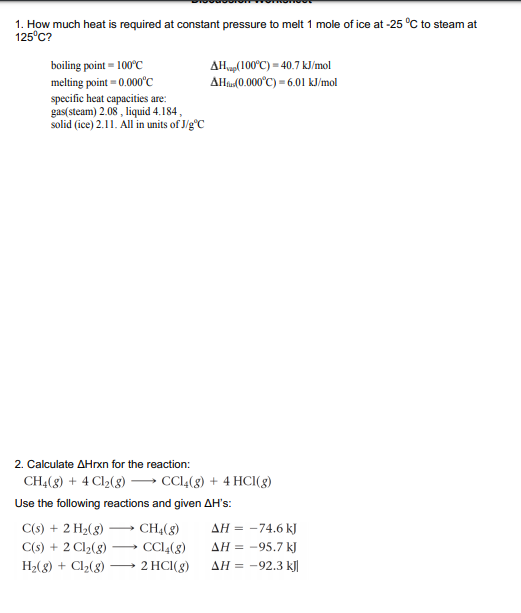1. How much heat is required at constant pressure to melt 1 mole of ice at -25 C to steam at 125° C? boiling point 100c melting point 0.000'C specific heat capacities are gas(steam) 2.08, liquid 4.184 solid (ice) 2.11. All in units of JigC AH(100°C) 40.7 kJ/mol AHu (0.000°C)-6.01 kJ/mol 2. Calculate AHrxn for the reaction: CH4(g)4 Cl2(g) -CC4(g) 4 HCl (g) + Use the following reactions and given AH's: CH4(g) AH =-74.6 kJ 2 H2(g) C(s) C(s)2 Cl2(g)...

Free Homework App